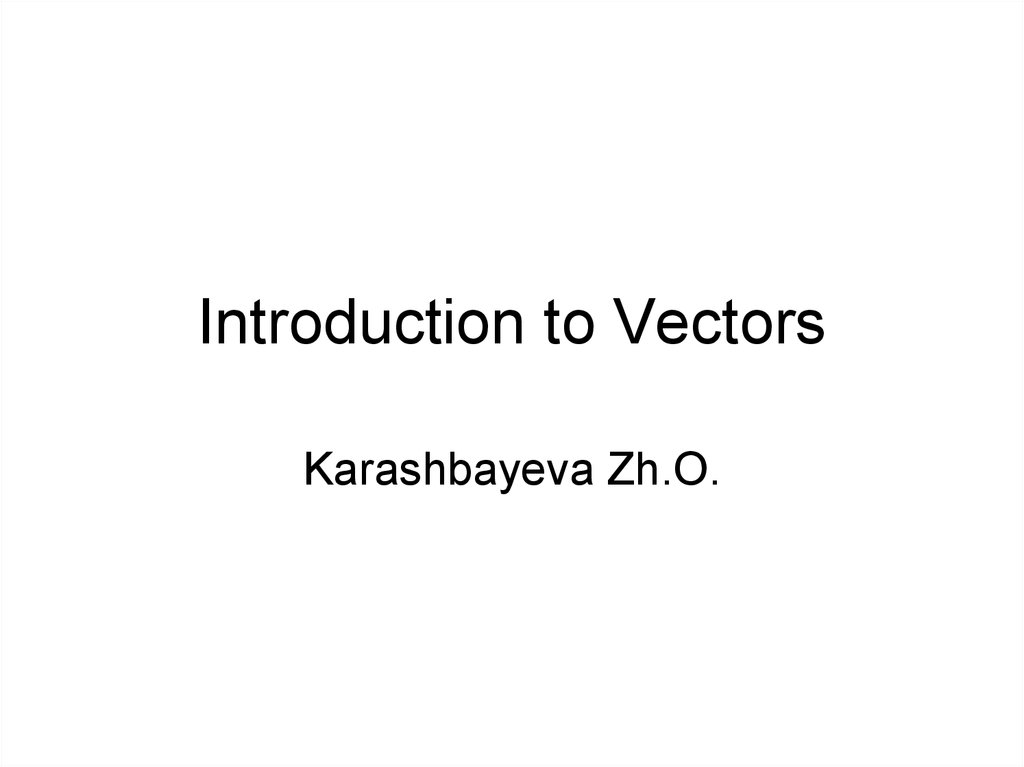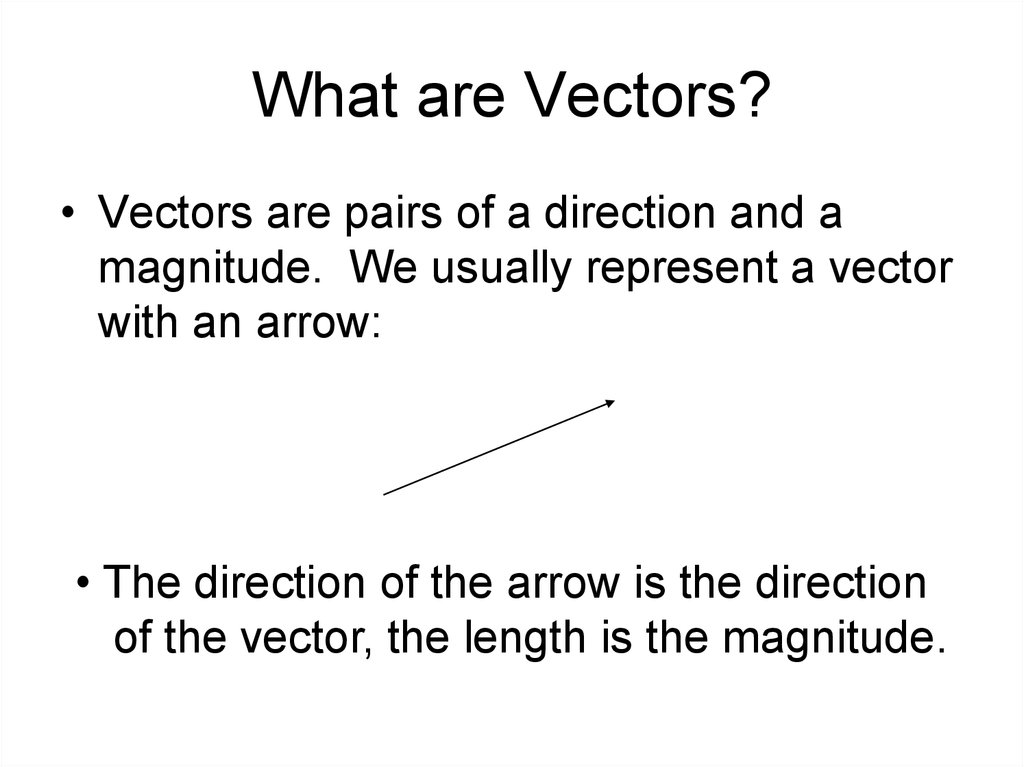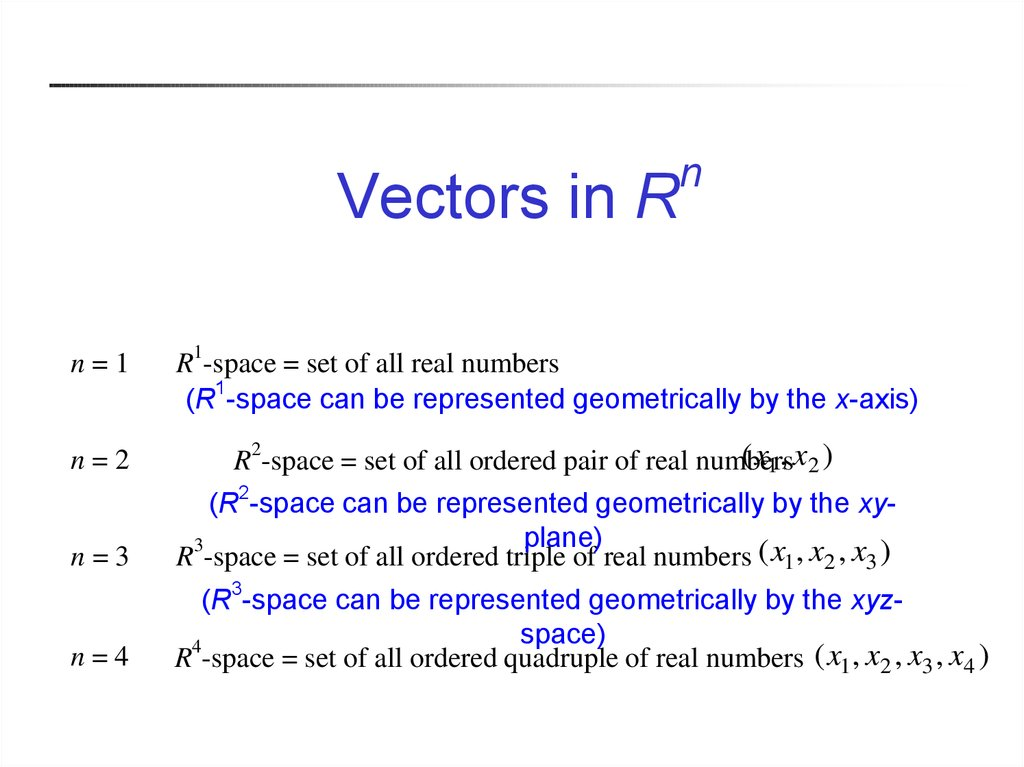# Introduction to vectors

## 1. Introduction to Vectors

Karashbayeva Zh.O.

## 2. What are Vectors?

• Vectors are pairs of a direction and a
magnitude. We usually represent a vector
with an arrow:
• The direction of the arrow is the direction
of the vector, the length is the magnitude.

## 3. Vectors in Rn

Vectors in R
n=1
n
R1-space = set of all real numbers
(R1-space can be represented geometrically by the x-axis)
n=2
( x1 , x2 )
R2-space = set of all ordered pair of real numbers
n=3
(R2-space can be represented geometrically by the xyplane)
R3-space = set of all ordered triple of real numbers ( x1 , x2 , x3 )
n=4
(R3-space can be represented geometrically by the xyzspace)
4
R -space = set of all ordered quadruple of real numbers ( x1 , x2 , x3 , x4 )

## 4. Multiples of Vectors

Given a real number c, we can multiply a
vector by c by multiplying its magnitude by
c:
2v
v
-2v
Notice that multiplying a vector by a
negative real number reverses the direction.

Two vectors can be added using the
Parallelogram Law
u
u+v
v

## 6. Combinations

These operations can be combined.
2u
2u - v
u
v
-v

## 7. Components

To do computations with vectors, we place
them in the plane and find their
components.
v
(2,2)
(5,6)

## 8. Components

The initial point is the tail, the head is the
terminal point. The components are
obtained by subtracting coordinates of the
initial point from those of the terminal
(5,6)
point.
v
(2,2)

## 9. Components

The first component of v is 5 -2 = 3.
The second is 6 -2 = 4.
We write v = <3,4>
v
(2,2)
(5,6)

## 10. Magnitude

The magnitude of the vector is the length
of the segment, it is written ||v||.
v
(2,2)
(5,6)

## 11. Scalar Multiplication

Once we have a vector in component
form, the arithmetic operations are easy.
To multiply a vector by a real number,
simply multiply each component by that
number.
Example: If v = <3,4>, -2v = <-6,-8>

components.
For example, if v = <3,4> and w = <-2,5>,
then v + w = <1,9>.
Other combinations are possible.
For example: 4v – 2w = <16,6>.

## 13. Unit Vectors

A unit vector is a vector with magnitude 1.
Given a vector v, we can form a unit vector
by multiplying the vector by 1/||v||.
For example, find the unit vector in the
direction <3,4>:

## 14. Special Unit Vectors

A vector such as <3,4> can be written as
3<1,0> + 4<0,1>.
For this reason, these vectors are given
special names: i = <1,0> and j = <0,1>.
A vector in component form v = <a,b> can
be written ai + bj.

## 17. Spanning Sets and Linear Independence

Linear combination :
A vector u in a vector space V is called a linear combination of
the vectors v1 , v 2 ,
, v k in V if u can be written in the form
u c1v1 c2 v 2
where c1 , c2 ,
ck v k ,
, ck are real-number scalars

## 18.

Ex : Finding a linear combination
v1 (1,2,3) v 2 (0,1,2) v 3 ( 1,0,1)
Prove (a) w (1,1,1) is a linear combination of v1 , v 2 , v 3
(b) w (1, 2,2) is not a linear combination of v1 , v 2 , v 3
Sol:
(a) w c1v1 c2 v 2 c3 v3
1,1,1 c1 1,2,3 c2 0,1,2 c3 1,0,1
(c1 c3 , 2c1 c2 , 3c1 2c2 c3 )
c1
c3
2c1 c2
3c1 2c2 c3
1
1
1

## 19.

1 0 1 1
G.-J. E.
2 1 0 1
3 2 1 1
1 0 1 1
0 1 2 1
0 0 0 0
c1 1 t , c2 1 2t , c3 t
(this system has infinitely many solutions)
t 1
w 2 v1 3 v 2 v 3
t 2
w 3v1 5v 2 2 v 3

## 20.

(b)
w c1 v1 c2 v 2 c3 v 3
1 0 1 1
G.-J. E.
2 1 0 2
3 2 1
2
1 0 1 1
0 1 2 4
0 0 0
7
This system has no solution since the third row means
0 c1 0 c2 0 c3 7
w can not be expressed as c1v1 c2 v2 c3 v3

## 21.

The
span of a set: span(S)
If S={v1, v2,…, vk} is a set of vectors in a vector space V,
then the span of S is the set of all linear combinations of
the vectors in S,
span(S ) c1v1 c2 v 2
ck v k
ci R
(the set of all linear combinations of vectors in S )
Definition of a spanning set of a vector space:
If every vector in a given vector space V can be written as a
linear combination of vectors in a set S, then S is called a
spanning set of the vector space V

## 22.

Note: The above statement can be expressed as follows
span( S ) V
S spans (generates) V
V is spanned (generated) by S
S is a spanning set of V
Ex 4:
(a) The set S {(1, 0, 0), (0,1, 0), (0, 0,1)} spans R3 because any vector
u (u1 , u2 , u3 ) in R3 can be written as
u u1 (1, 0, 0) u2 (0,1, 0) u3 (0, 0,1)
(b) The set S {1, x, x 2 } spans P2 because any polynomial function
p( x) a bx cx 2 in P2 can be written as
p( x) a(1) b( x) c( x 2 )

## 23.

Ex 5: A spanning set for R3
Show that the set S (1,2,3),(0,1,2),( 2,0,1) spans R3
Sol:
We must determine whether an arbitrary vector u (u1 , u2 ,u3 )
in R 3 can be expressed as a linear combination of v1 =(1,2,3),
v 2 =(0,1,2), and v 3 ( 2, 0,1)
If u c1v1 c2 v 2 c3 v3 c1
2c3 u1
2c1 c2
u2
3c1 2c2 c3 u3
The above problem thus reduces to determining whether this
system is consistent for all values of u1 , u2 , and u3

## 24.

1 0 2
A 2 1
3 2
0 0
1
Ax u has exactly one solution for every u
span( S ) R3

## 25.

Definitions
of Linear Independence (L.I.) and Linear Dependence
(L.D.) :
S v1 , v2 ,
For c1v1 c2 v 2
, vk : a set of vectors in a vector space V
ck v k 0
(1) If the equation has only the trivial solution (c1 c2
then S (or v1 , v 2 ,
ck 0)
, v k ) is called linearly independent
(2) If the equation has a nontrivial solution (i.e., not all zeros),
then S (or v1 , v 2 ,
, v k ) is called linearly dependent (The name of
linear dependence is from the fact that in this case, there exist a v i
which can be represented by the linear combination of {v1 , v 2 ,
v i 1 ,
v k } in which the coefficients are not all zero.
, v i 1 ,

## 26.

Ex : Testing for linear independence
Determine whether the following set of vectors in R3 is L.I. or L.D.
S v1 , v 2 , v 3 1, 2, 3 , 0, 1, 2 , 2, 0, 1
Sol:
c1
c1v1 c2 v 2 c3 v 3 0
2c3 0
2c1 c2
0
3c1 2c2 c3 0
1 0 2 0
1 0 0 0
0 1 0 0
G.-J. E.
2 1 0 0
3 2 1 0
0 0 1 0
c1 c2 c3 0 only the trivial solution
(or det(A) 1 0, so there is only the trivial solution)
S is (or v1, v 2 , v3 are) linearly independent

## 27.

• EX: Testing for linear independence
Determine whether the following set of vectors in P2 is L.I. or L.D.
S v1 , v 2 , v 3 1 x 2 x 2 ,2 5 x x 2 ,x x 2
Sol:
c1v1+c2v2+c3v3 = 0
i.e., c1(1+x – 2x2) + c2(2+5x – x2) + c3(x+x2) = 0+0x+0x2
c1+2c2
=0
c1+5c2+c3 = 0
–2c1– c2+c3 = 0
1 2
1 2 0 0
G. E.
1 5 1 0
0 1
2 1 1 0
0 0
0
1
0
3
0 0
0
This system has infinitely many solutions
(i.e., this system has nontrivial solutions, e.g., c1=2, c2= – 1, c3=3)
S is (or v1, v2, v3 are) linearly dependent

## 28. Basis and Dimension

• Basis :
Basis and Dimension
Spanning
Sets
V: a vector space
S ={v1, v2, …, vn} V
(1)
(2)
Bases
Linearly
Independent
Sets
S spans V (i.e., span(S) = V)
(For any u V ,
V
c v =Ax u has a solution (det(A) 0),
i
i
see Ex 5 on Slide 4.44)
S is linearly independent
(For ci vi = Ax 0, there is only the trivial solution (det(A) 0),
S is called a basis for V
Notes:
A basis S must have enough vectors to span V, but not so
many vectors that one of them could be written as a linear
combination of the other vectors in S

## 29.

Notes:
(1) the standard basis for R3:
{i, j, k} i = (1, 0, 0), j = (0, 1, 0), k = (0, 0, 1)
n
(2) the standard basis for R :
{e1, e2, …, en} e1=(1,0,…,0), e2=(0,1,…,0),…, en=(0,0,…,1)
Ex: For R4, {(1,0,0,0), (0,1,0,0), (0,0,1,0), (0,0,0,1)}

## 30.

(3) the standard basis matrix space:
Ex: 2 2 matrix space:
1 0 0 1 0 0 0 0
,
,
,
0 0 0 0 1 0 0 1
(4) the standard basis for Pn(x):
{1, x, x2, …, xn}
Ex: P3(x)
{1, x, x2, x3}

## 31.

Ex 2: The nonstandard basis for R2
Show that S ={v1 ,v 2 }={(1,1), (1, 1)} is a basis for R 2
c1 c2 =u1
(1) For any u=(u1 ,u 2 ) R , c1v1 +c2 v 2 =u
c1 c2 =u 2
2
Because the coefficient matrix of this system has a nonzero determinant, the
system has a unique solution for each u. Thus you can conclude that S spans R2
c1 c2 =0
(2) For c1v1 +c2 v 2 =0
c1 c2 =0
Because the coefficient matrix of this system has a nonzero determinant, you
know that the system has only the trivial solution. Thus you can conclude that S is
linearly independent
According to the above two arguments, we can conclude that S
is a (nonstandard) basis for R2